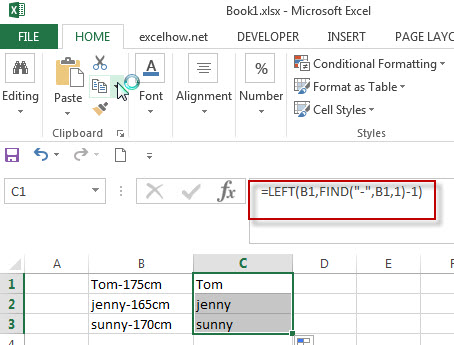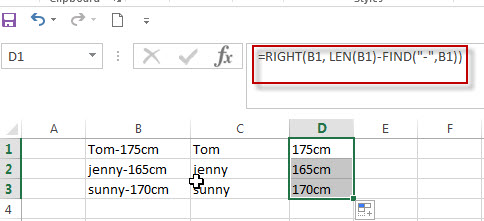# Split Text String by Specified Character in Excel

This post explains how to split text string by a specified character (such as: comma, space, dash, slash, etc.) using FIND function within other functions in excel. And you will learn that how to extract part of characters to the left of a specified character in one cell.

## Split Text String by Specified Character

When you want to split text string by a specified character, such as:  dash character, the most important thing is that how to get the position of the specified character (dash “-”) within the text string in one cell. So you can use the FIND function or SEARCH Function to get the position of the searched character within another string. Once you got the position number of the specified character, you can use the LEFT function to extract all the characters to the left of dash character (“-“).

So you should use the Left function in combination with FIND function to split the text string in excel. And we can refer to the below formula that will find the position of “-“in Cell B1 and extract all the characters to the left of the dash character “-“.

`=LEFT(B1,FIND("-",B1,1)-1)`

Suppose you have a list of student’s height in column B and you want to extract only the name part. You can use the above excel formula in Cell C1.In the above example, the FIND function will locate the position of the first dash character (“-“) in Cell B1, and then then LEFT function will use the returned position number of FIND function as the second argument to extract all the characters left (student’s name) to it.

The returned value of FIND function need to subtract 1, as we need to exclude the dash character “-“from the result of the LEFT function.

Of course, if you want to extract all characters to the right of dash character within the text string in Cell B1, you can use a combination of the RIGHT, LEN and FIND functions in excel.  Enter the below excel formula in Cell D1:

`=RIGHT(B1, LEN(B1)-FIND("-",B1))`In the above example, the FIND function will locate the position of the dash character in Cell B1. And the LEN Function will get the length of the text string in Cell B1. Then subtract the result of FIND function from the returned value of LEN function, so that it will get the number of characters from the rightmost character in Cell B1.

### Related Formulas

• Split Multiple Lines from a Cell into Rows
If you have multiple lines in a cell and each line is separated by line break character or press “alt + enter” key while you entering the text string into cells, and you need to extract the multiple lines into the separated rows or columns, you can use a combination with the TRIM function, the MID function, the SUBSTITUTE function, the REPT function, the LEN function to create a complex excel formula..…
• Split Text String to an Array
If you want to convert a text string into an array that split each character in text as an element, you can use an excel formula to achieve this result. the below will guide you how to use a combination of the MID function, the ROW function, the INDIRECT function and the LEN function to split a string…
• Split Text String by Line Break in Excel
When you want to split text string by line break in excel, you should use a combination of the LEFT, RIGHT, CHAR, LEN and FIND functions. The CHAR (10) function will return the line break character, the FIND function will use the returned value of the CHAR function as the first argument to locate the position of the line break character within the cell B1.…
• Split Text and Numbers
If you want to split text and numbers, you can run the following excel formula that use the MIN function, FIND function and the LEN function within the LEFT function in excel. And if you want to extract only numbers within string, then you also need to RIGHT function to combine with the above functions..…

### Related Functions

• Excel Find function
The Excel FIND function returns the position of the first text string (substring) from the first character of the second text string.The FIND function is a build-in function in Microsoft Excel and it is categorized as a Text Function.The syntax of the FIND function is as below:= FIND  (find_text, within_text,[start_num])…
• Excel SEARCH function
The Excel SEARCH function returns the number of the starting location of a substring in a text string.The syntax of the SEARCH function is as below:= SEARCH  (find_text, within_text,[start_num])…
• Excel LEFT function
The Excel LEFT function returns a substring (a specified number of the characters) from a text string, starting from the leftmost character.The LEFT function is a build-in function in Microsoft Excel and it is categorized as a Text Function.The syntax of the LEFT function is as below:= LEFT(text,[num_chars])…
• Excel LEN function
The Excel LEN function returns the length of a text string (the number of characters in a text string).The LEN function is a build-in function in Microsoft Excel and it is categorized as a Text Function.The syntax of the LEN function is as below:= LEN(text)…
• Excel RIGHT function
The Excel RIGHT function returns a substring (a specified number of the characters) from a text string, starting from the rightmost character.The syntax of the RIGHT function is as below:= RIGHT (text,[num_chars])…